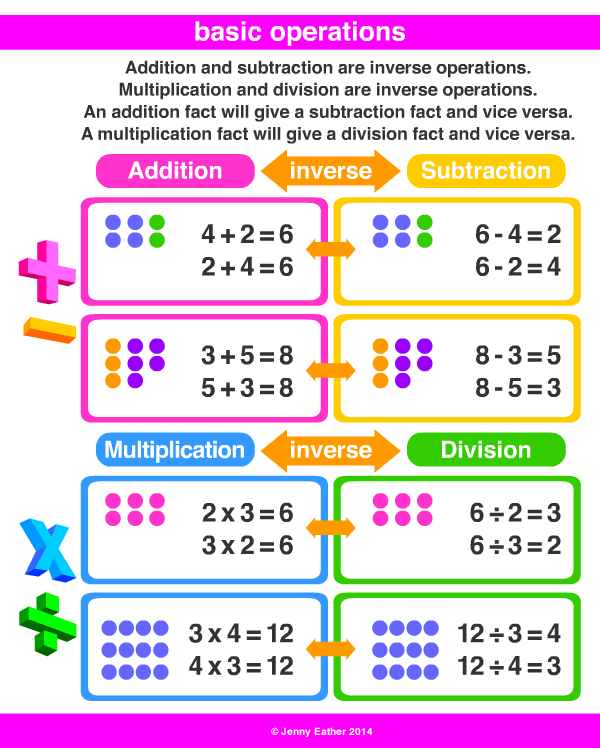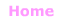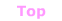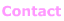Aa Bb Cc Dd Ee Ff Gg Hh Ii Jj Kk Ll Mm Nn Oo Pp Qq Rr Ss Tt Uu Vv Ww Xx Yy Zz

Oo

operations

• mathematical procedures or processes
used to work something out.

EXAMPLES:common operations

Subtraction (−)
minuend − subtrahend = difference

Multiplication (×)
multiplicand × multiplier = product

Division (÷)
dividend ÷ divisor = quotient

Exponentiation
baseexponent = power

nth root (√)© Jenny Eather 2014. All rights reserved.